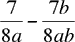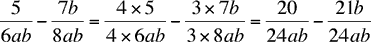Quandaries and Queries Who is asking: Student Level: All Question: _5_ - __7__ 6ab      8a Hi Filipe, To add or subtract two fractions you need the denominators to be the same. The denominator of the first fraction is 6ab and the denominator of the second is 8a. You can transform the second fraction into an equivalent fraction with 8ab in the denominator by multiplying the numerator and denominator of the second fraction by b. That isNow the denominators are 6ab and 8ab. 24 is a multiple of 8 and 6 so you can make both denominators 24ab by multiplying the numerator and demoninator of the first equation by 4 and the numerator and denominator of the second equation by 3. That isPenny Go to Math Central